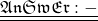Question

3. A large crane lifts a 25,000 kg mass in the air. The amount of work that must be done by the
crane’s lifting components is 2.2 X 10’J (Wo). If the efficiency of the crane is 22%, how much
useful work is done on the mass (Wou)?

1.Actually Welcome to the concept of Efficiency.

Here we can see that, the Input work is given as 2.2 x 10^7 J and the efficiency is given as 22%

The efficiency is => 22% => 22/100.

so we get as,

E = W(output) /W(input)

hence, W(output) = E x W(input)

so we get as,

W(output) = (22/100) x 2.2 x 10^7

=> W(output) = 0.22 x 2.2 x 10^7 => 0.484 x 10^7

hence, W(output) = 4.84 x 10^6 J

The useful work done on the mass is 4.84 x 10^6 J